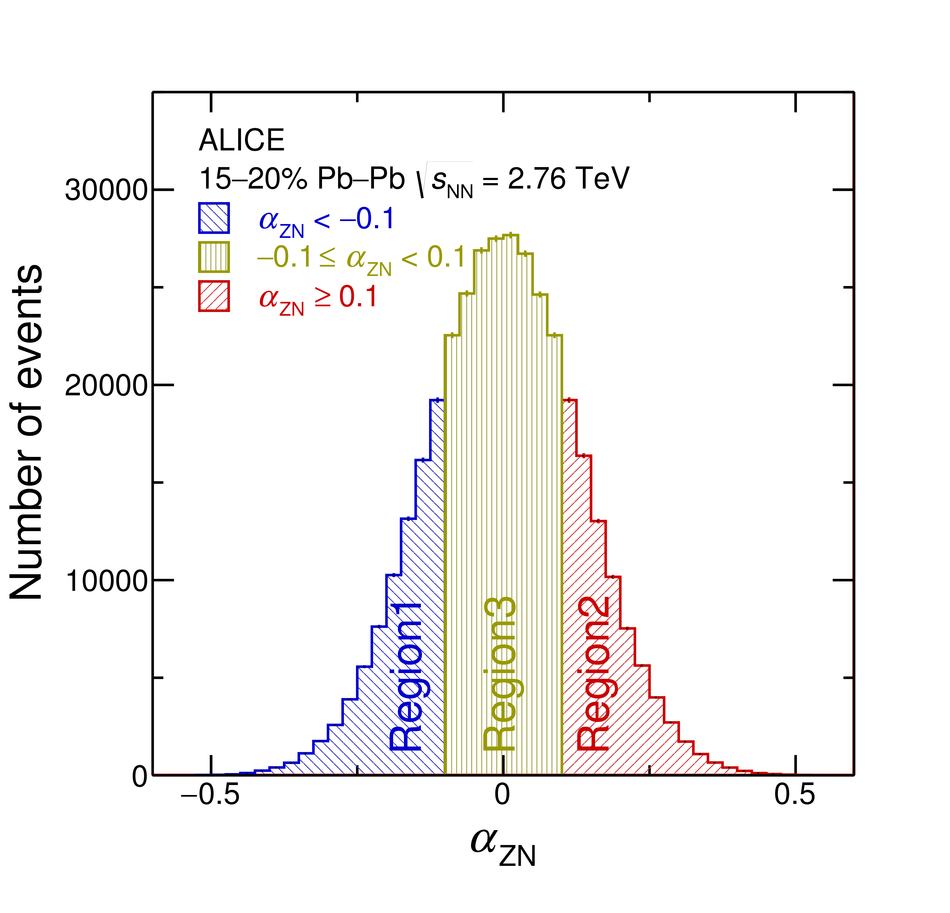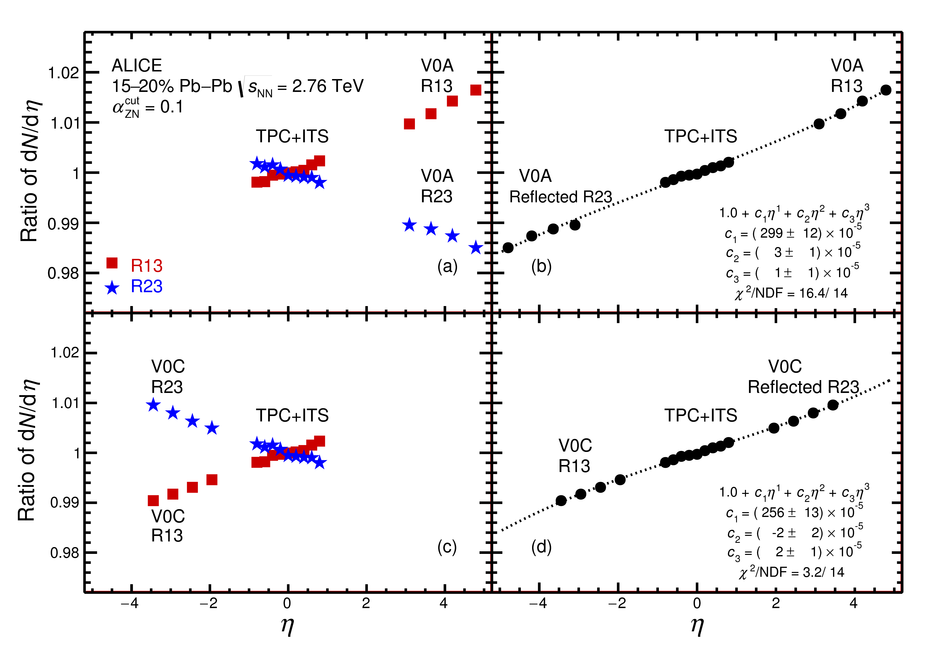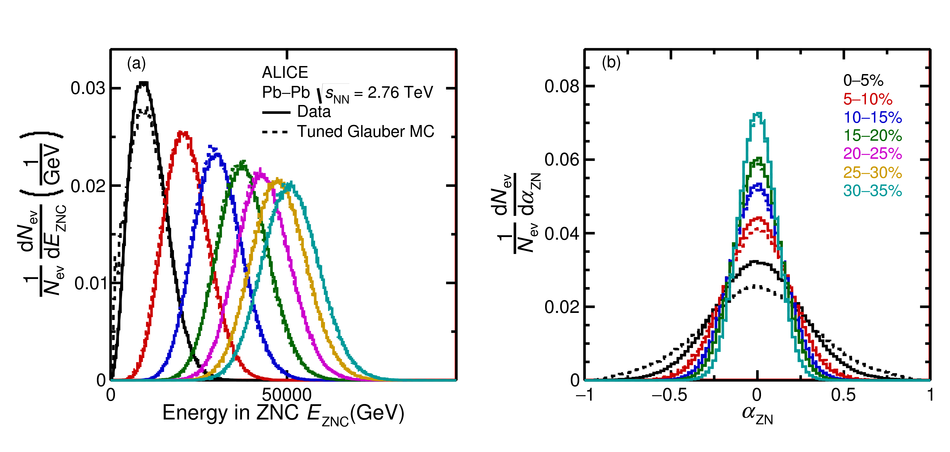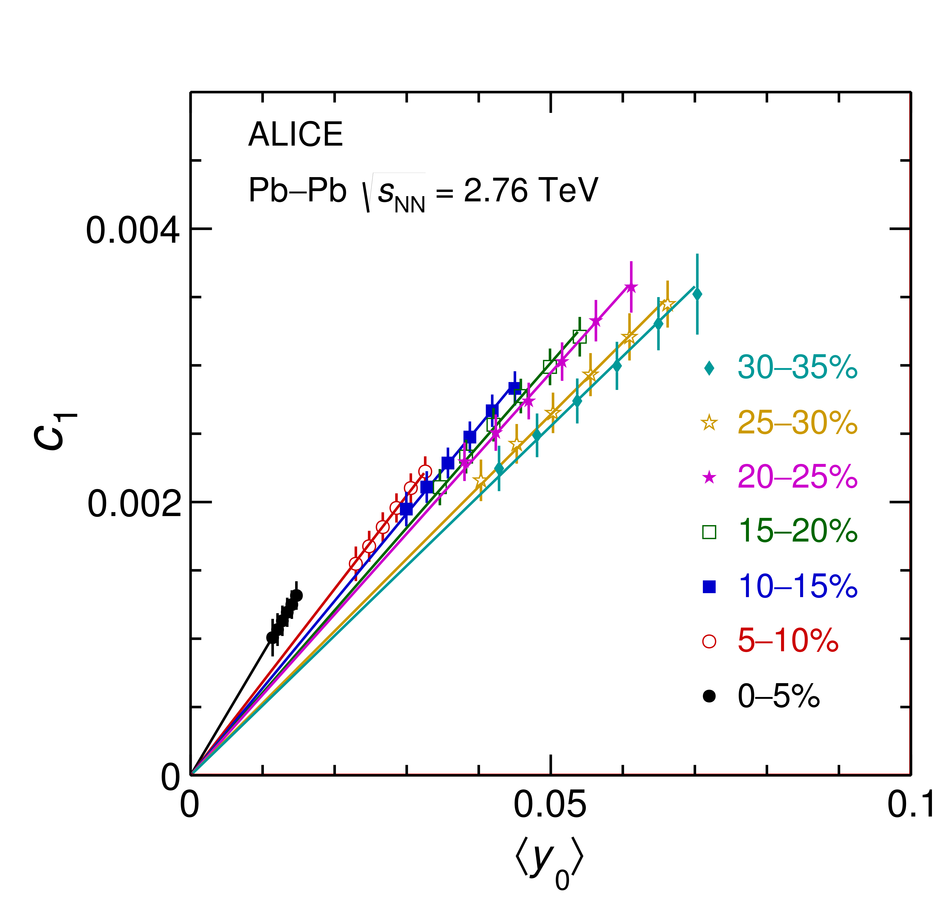# Longitudinal asymmetry and its effect on pseudorapidity distributions in Pb-Pb collisions at $\sqrt{s_{NN}}$ = 2.76 TeV

First results on the longitudinal asymmetry and its effect on the pseudorapidity distributions in Pb-Pb collisions at $\sqrt{s_{\mathrm{NN}}}$ = 2.76 TeV at the Large Hadron Collider are obtained with the ALICE detector. The longitudinal asymmetry arises because of an unequal number of participating nucleons from the two colliding nuclei, and is estimated for each event by measuring the energy in the forward neutron-Zero-Degree-Calorimeters (ZNs). The effect of the longitudinal asymmetry is measured on the pseudorapidity distributions of charged particles in the regions $|\eta| <~ 0.9$, $2.8 <~ \eta <~ 5.1$ and $-3.7 <~ \eta <~ -1.7$ by taking the ratio of the pseudorapidity distributions from events corresponding to different regions of asymmetry. The coefficients of a polynomial fit to the ratio characterise the effect of the asymmetry. A Monte Carlo simulation using a Glauber model for the colliding nuclei is tuned to reproduce the spectrum in the ZNs and provides a relation between the measurable longitudinal asymmetry and the shift in the rapidity ($y_{\mathrm{0}}$) of the participant zone formed by the unequal number of participating nucleons. The dependence of the coefficient of the linear term in the polynomial expansion, $c_{\rm 1}$, on the mean value of $y_{\mathrm{0}}$ is investigated.

Figures

## Figure 1

 The distribution of the asymmetry parameter $\alpha_{\rm{ZN}}$ for the 15--20\% centrality interval. The distribution is demarcated into three regions using $|\alpha_{\rm {ZN}}^{\rm {cut}}|$. A Gaussian fit to the distribution yields a width of 0.13.## Figure 2

 The ratio of $\dNdeta$ distribution for events from the different regions of $\alpha_{\rm{ZN}}$ distribution of Fig.~\ref{fig:AlphaDisbnData}. The $\dNdeta$ distributions areobtained as described in Sect.~\ref{sec:expdetail}. (a) The square (star) symbols corresponding to R13 (R23) are obtained by taking the ratio of $\dNdeta$ of events from Region 1 (Region 2) to Region 3. (b) The data points are obtained after reflection across $\eta = 0$ as described in the text The data for $|\eta| > 1.0$ in panels (a) and (b) are frommeasurements in V0A and in panels (c) and (d) are from measurements inV0C.## Figure 3

 The coefficient $c_{\rm 1}$ characterising the change in $\dNdeta$ distribution for asymmetric regions is shown for different values of $\alpha_{\rm{ZN}}^{\rm{cut}}$ ($\alpha_{\rm{ZN}}^{\rm{cut}}$ demarcates the asymmetric and symmetric events) for each centrality interval.## Figure 4

 Rapidity-shift $y_{\rm 0}$ as a function of asymmetry in (a) number of participants (b) number of spectators (c) number of spectator neutrons and (d) energy in ZN obtained using TGMC as described in the text. Theresults in all four panels are shown for the 15--20\% centrality interval.## Figure 5

 (a) Distribution of energy in ZNC in each 5\% centrality interval for events simulated using TGMC and for the experimental data. (b) Distribution of the asymmetry parameter $\alpha_{\rm{ZN}}$ in the simulated events and in experimental data for different centralities. For clarity, only 5 distributions are shown. The distributions corresponding to 20-25\% and 25-30\% lie between those of 15-20\% and 30-35\%.## Figure 6

 The distribution of rapidity-shifts for the events from the three different regions of measured asymmetry shown in Fig.~\ref{fig:AlphaDisbnData}. Determination of $y_{\rm 0}$ uses the difference in number of nucleons. For small values of this difference, the changes in values near $y_0 = 0$ are discrete, and are smeared into a continuous distribution as $y_{\rm 0}$ increases.## Figure 7

 (a) The estimated mean value of rapidity-shift $\langle y_{\rm 0} \rangle$ for the asymmetric region characterised by different values of $\alpha_{\rm{ZN}}^{\rm{cut}}$ for each centrality interval. (b) The coefficient $c_{\rm 1}$ characterising the change in the pseudorapidity distributions for different values of $\alpha_{\rm{ZN}}^{\rm{cut}}$, for each centrality interval. These results are obtained using TGMC and simulated pseudorapidity distributions, as described in the text## Figure 8

 The mean values of the coefficient $c_{\rm 1}$ are shown as filled (red) circles for different centralities. These correspond to the ratio of $\dNdeta$ distributions of populations of events demarcated by $\alpha_{\rm{ZN}}^{\rm{cut}}$ = 0.1. The squares show the corresponding values from simulations, and correspond to $\alpha_{\rm{ZN}}^{\rm{cut}}$ = 0.1 in Fig.~\ref{fig:Meany0vsAsym}, for different centralities. The systematic uncertainties are shown as bands.## Figure 9

 $\langle y_0 \rangle$